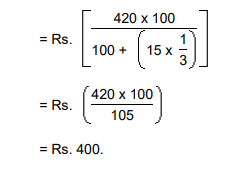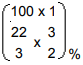## Banker’s Discount

### Suggested Lessons

Definition of banker's discount. The difference between the value shown on a bond, share etc that is bought by a bank from a customer, and the amount that the customer actually receives from the bank. The banker's discount is kept by the bank as payment. Suppose a merchant A buys goods worth, say Rs. 10,000 from another merchant B at a credit of say 5 months. Then, B prepares a bill, called the bill of exchange. A signs this bill and allows B to withdraw the amount from his bank account after exactly 5 months. The date exactly after 5 months is called nominally due date. Three days (known as grace days) are added to it get a date, known as legally due date. Suppose B wants to have the money before the legally due date. Then he can have the money from the banker or a broker, who deducts S.I. on the face vale (i.e., Rs. 10,000 in this case) for the period from the date on which the bill was discounted (i.e., paid by the banker) and the legally due date. This amount is know as Banker's Discount (B.D.). Thus, B.D. is the S.I. on the face value for the period from the date on which the bill was discounted and the legally due date. Banker's Gain (B.G.) = (B.D.) - (T.D.) for the unexpired time. Note: When the date of the bill is not given, grace days are not to be added. IMPORTANT FORMULAE 1. B.D. = S.I. on bill for unexpired time. 2. B.G. = (B.D.) - (T.D.) = S.I. on T.D. =3. T.D. P.W. x B.G. 4. B.D. =5. T.D. =6. Amount =7. T.D. =1. The banker's discount on a bill due 4 months hence at 15% is Rs. 420. The true discount is: A. Rs. 400 B. Rs. 360 C. Rs. 480 D. Rs. 320 Answer: Option A Explanation: T.D. =2. The banker's discount on Rs. 1600 at 15% per annum is the same as true discount on Rs. 1680 for the same time and at the same rate. The time is: A. 3 months B. 4 months C. 6 months D. 8 months Answer: Option B Explanation: S.I. on Rs. 1600 = T.D. on Rs. 1680. Rs. 1600 is the P.W. of Rs. 1680, i.e., Rs. 80 is on Rs. 1600 at 15%. Time == 4 months. 3. The banker's gain of a certain sum due 2 years hence at 10% per annum is Rs. 24. The present worth is: A. Rs. 480 B. Rs. 520 C. Rs. 600 D. Rs. 960 Answer: Option C Explanation: T.D. == Rs.= Rs. 120. P.W. == Rs.= Rs. 600. 4. The banker's discount on a sum of money foryears is Rs. 558 and the true discount on the same sum for 2 years is Rs. 600. The rate percent is: A. 10% B. 13% C. 12% D. 15% Answer: Option C Explanation: B.D. foryears = Rs. 558. B.D. for 2 years = Rs.= Rs. 744 T.D. for 2 years = Rs. 600. Sum == Rs.= Rs. 3100. Thus, Rs. 744 is S.I. on Rs. 3100 for 2 years. Rate == 12%   5. The banker's gain on a sum due 3 years hence at 12% per annum is Rs. 270. The banker's discount is: A. Rs. 960 B. Rs. 840 C. Rs. 1020 D. Rs. 760 Answer: Option C Explanation: T.D. == Rs.= Rs. 750. B.D. = Rs.(750 + 270) = Rs. 1020. 6. The banker's discount of a certain sum of money is Rs. 72 and the true discount on the same sum for the same time is Rs. 60. The sum due is: A. Rs. 360 B. Rs. 432 C. Rs. 540 D. Rs. 1080 Answer: Option A Explanation: Sum == Rs.= Rs.= Rs. 360.   7. The certain worth of a certain sum due sometime hence is Rs. 1600 and the true discount is Rs. 160. The banker's gain is: A. Rs. 20 B. Rs. 24 C. Rs. 16 D. Rs. 12 Answer: Option C Explanation: B.G. == Rs.= Rs. 16. 8. The present worth of a certain bill due sometime hence is Rs. 800 and the true discount is Rs. 36. The banker's discount is: A. Rs. 37 B. Rs. 37.62 C. Rs. 34.38 D. Rs. 38.98 Answer: Option B Explanation: B.G. == Rs.= Rs. 1.62 ∴ B.D. = (T.D. + B.G.) = Rs. (36 + 1.62) = Rs. 37.62 (Ans)   9. The banker's gain on a bill due 1 year hence at 12% per annum is Rs. 6. The true discount is: A. Rs. 72 B. Rs. 36 C. Rs. 54 D. Rs. 50 Answer: Option D Explanation: T.D. == Rs.= Rs. 50.(Ans)   10. The banker's gain on a certain sum dueyears hence isof the banker's discount. The rate percent is: A.B.C.D.Answer: Option B Explanation: Let, B.D = Re. 1. Then, B.G. = Re.∴ T.D. = (B.D. - B.G.) = Re.= Re.Sum == Rs.S.I. on Rs.foryears is Re. 1. ∴ Rate =11. The present worth of a sum due sometime hence is Rs. 576 and the banker's gain is Rs. 16. The true discount is: A. Rs. 36 B. Rs. 72 C. Rs. 48 D. Rs. 96 Answer: Option D Explanation: T.D. = P.W. x B.G. = 576 x 16 = 96. 12. The true discount on a bill of Rs. 540 is Rs. 90. The banker's discount is: A. Rs. 60 B. Rs. 108 C. Rs. 110 D. Rs. 112 Answer: Option B Explanation: P.W. = Rs. (540 - 90) = Rs. 450. S.I. on Rs. 450 = Rs. 90. S.I. on Rs. 540 = Rs.= Rs. 108. B.D. = Rs. 108. 13. The banker's discount on a certain sum due 2 years hence isof the true discount. The rate percent is: A. 11% B. 10% C. 5% D. 5.5% Answer: Option C Explanation: Let T.D. be Re. 1. Then, B.D. = Rs.= Rs. 1.10. ∴ Sum = Rs.= Rs.= Rs. 11. ∴ S.I. on Rs. 11 for 2 years is Rs. 1.10 ∴ Rate == 5%.